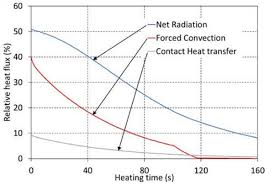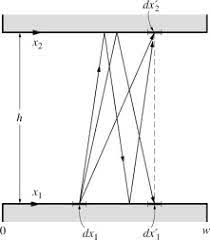## How to Calculate and Solve for Net Heat Flux for Radiation | Radiation Heat TransferThe image above represents net heat flux for radiation.

To compute for net heat flux for radiation, four essential parameters are needed and these parameters are Emissivity (ε), Area (A), Emitting Power of Black Body (eb) and Radiosity (J).

The formula for calculating net heat flux for radiation:

qnet = εAeb / 1 – ε – J

Where:

qnet = Net Heat Flux for Radiation
ε = Emissivity
A = Area
eb = Emitting Power of Black Body

Let’s solve an example;
Find the net heat flux for radiation when the emissivity is 22, the area is 2, the emitting power of black body is 10 and the radiosity is 14.

This implies that;

ε = Emissivity = 22
A = Area = 2
eb = Emitting Power of Black Body = 10

qnet = εAeb / 1 – ε – J
qnet = (22)(2)(10) / 1 – 22 – 14
qnet = 440 / -21 – 14
qnet = -20.9523 – 14
qnet = -34.95

Therefore, the net heat flux for radiation is -34.95 W.

## How to Calculate and Solve for Radiosity | Radiation Heat TransferTo compute for radiosity, three essential parameters are needed and these parameters are Total Emissive Power (e), Reflectivity (ρ) and Total Irradiation (G).

J = e + ρG

Where:

e = Total Emissive Power
ρ = Reflectivity

Let’s solve an example;
Find the radiation heat transfer when the total emissive power is 12, the reflectivity is 10 and the total irradiation is 20.

This implies that;

e = Total Emissive Power = 12
ρ = Reflectivity = 10
G = Total Irradiation = 20

J = e + ρG
J = 12 + (10)(20)
J = 12 + 200
J = 212

Therefore, the radiosity is 212 W/m².

Calculating the Total Emissive Power when the Radiosity, the Reflectivity and the Total Irradiation is Given.

e = J – ρG

Where:

e = Total Emissive Power
ρ = Reflectivity

Let’s solve an example;
Find the total emissive power when the radiation heat transfer is 32, the reflectivity is 10 and the total irradiation is 2.

This implies that;

J = Radiation Heat Transfer = 32
ρ = Reflectivity = 10
G = Total Irradiation = 2

e = J – ρG
e = 32 – (10)(2)
e = 32 – 20
e = 12

Therefore, the total emissive power is 12.